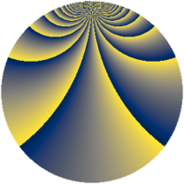# Properties

 Label 1600.2.crLevel $1600$ Weight $2$ Character orbit 1600.cr Rep. character $\chi_{1600}(21,\cdot)$ Character field $\Q(\zeta_{80})$ Dimension $7616$ Sturm bound $480$

# Related objects

## Defining parameters

 Level: $$N$$ $$=$$ $$1600 = 2^{6} \cdot 5^{2}$$ Weight: $$k$$ $$=$$ $$2$$ Character orbit: $$[\chi]$$ $$=$$ 1600.cr (of order $$80$$ and degree $$32$$) Character conductor: $$\operatorname{cond}(\chi)$$ $$=$$ $$1600$$ Character field: $$\Q(\zeta_{80})$$ Sturm bound: $$480$$

## Dimensions

The following table gives the dimensions of various subspaces of $$M_{2}(1600, [\chi])$$.

Total New Old
Modular forms 7744 7744 0
Cusp forms 7616 7616 0
Eisenstein series 128 128 0

## Trace form

 $$7616q - 24q^{2} - 24q^{3} - 24q^{4} - 32q^{5} - 24q^{6} - 64q^{7} - 24q^{8} - 24q^{9} + O(q^{10})$$ $$7616q - 24q^{2} - 24q^{3} - 24q^{4} - 32q^{5} - 24q^{6} - 64q^{7} - 24q^{8} - 24q^{9} - 32q^{10} - 24q^{11} - 24q^{12} - 24q^{13} - 24q^{14} - 32q^{15} - 24q^{16} - 24q^{17} - 64q^{18} - 24q^{19} - 32q^{20} - 24q^{21} - 24q^{22} - 24q^{23} - 64q^{24} - 32q^{25} - 144q^{26} - 24q^{27} - 24q^{28} - 24q^{29} - 112q^{30} - 64q^{32} - 24q^{34} - 32q^{35} + 216q^{36} - 24q^{37} - 24q^{38} - 24q^{39} + 128q^{40} - 24q^{41} - 24q^{42} - 64q^{43} - 24q^{44} - 32q^{45} - 24q^{46} - 24q^{47} - 24q^{48} - 64q^{49} - 8q^{50} - 64q^{51} - 24q^{52} - 24q^{53} - 24q^{54} - 96q^{55} - 24q^{56} - 64q^{57} - 24q^{58} - 24q^{59} + 64q^{60} - 24q^{61} - 56q^{62} - 48q^{63} - 24q^{64} - 64q^{65} - 56q^{66} - 24q^{67} - 64q^{68} - 24q^{69} + 64q^{70} - 24q^{71} - 24q^{72} - 24q^{73} - 64q^{74} - 96q^{75} - 64q^{76} - 24q^{77} - 24q^{78} - 24q^{79} - 8q^{80} - 24q^{81} - 224q^{82} - 24q^{83} - 24q^{84} - 32q^{85} - 24q^{86} - 24q^{87} - 24q^{88} - 24q^{89} - 32q^{90} - 24q^{91} - 328q^{92} - 112q^{93} - 104q^{94} - 24q^{96} - 24q^{98} - 112q^{99} + O(q^{100})$$

## Decomposition of $$S_{2}^{\mathrm{new}}(1600, [\chi])$$ into newform subspaces

The newforms in this space have not yet been added to the LMFDB.